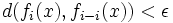# Homotopic maps are close

Suppose$X$ is a compact space and$(Y,d)$ is a metric space. Suppose$f$ and$g$ are homotopic maps from$X$ to$Y$. Then, there exists an$\epsilon > 0$ and a sequence of maps$f=f_0,f_1,f_2,\ldots,f_n = g$ such that for every$x \in X$:$d(f_i(x),f_{i-i}(x)) < \epsilon$
A related fact is that close maps are homotopic: the condition of compactness is now on$Y$ instead of on$X$.